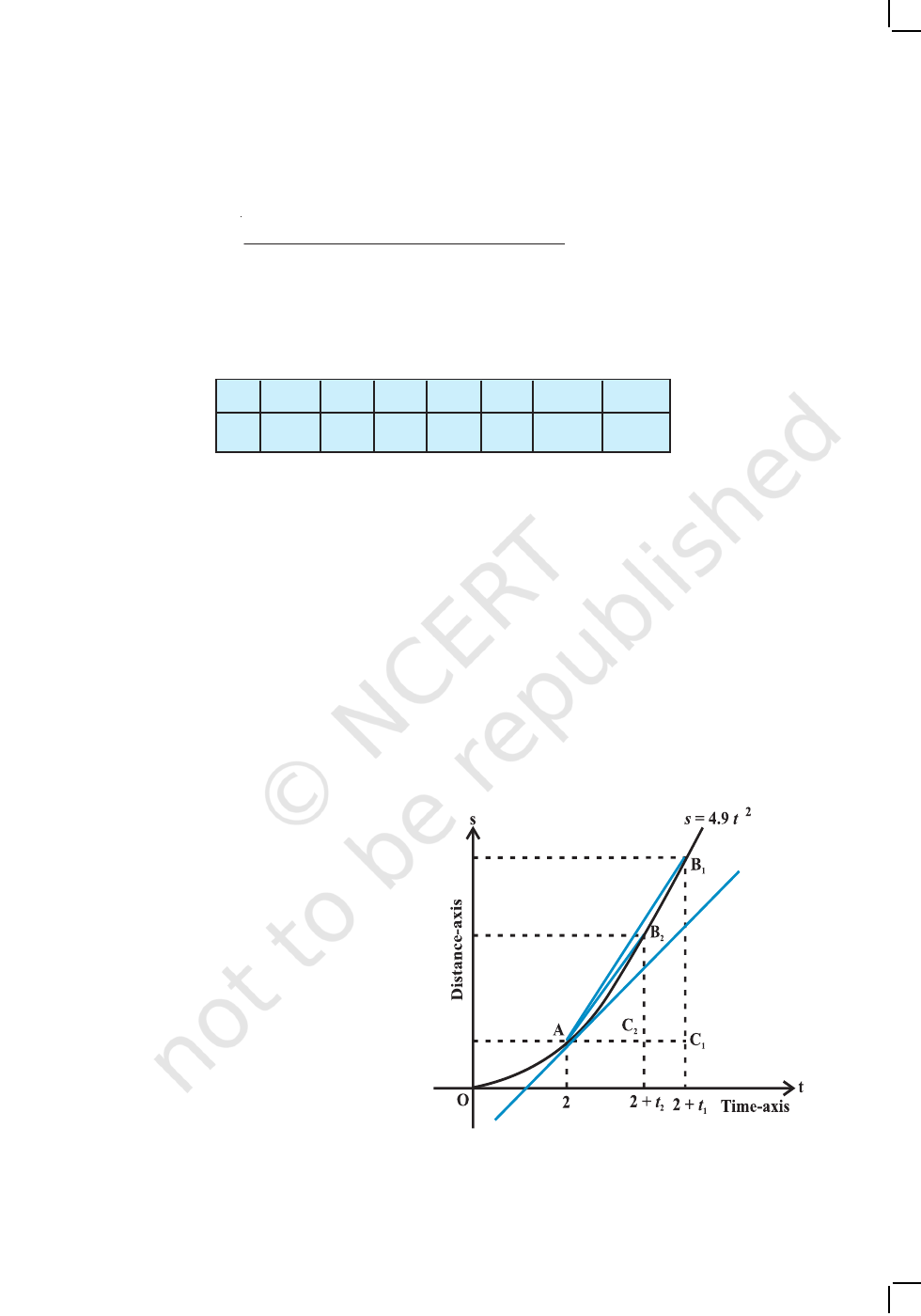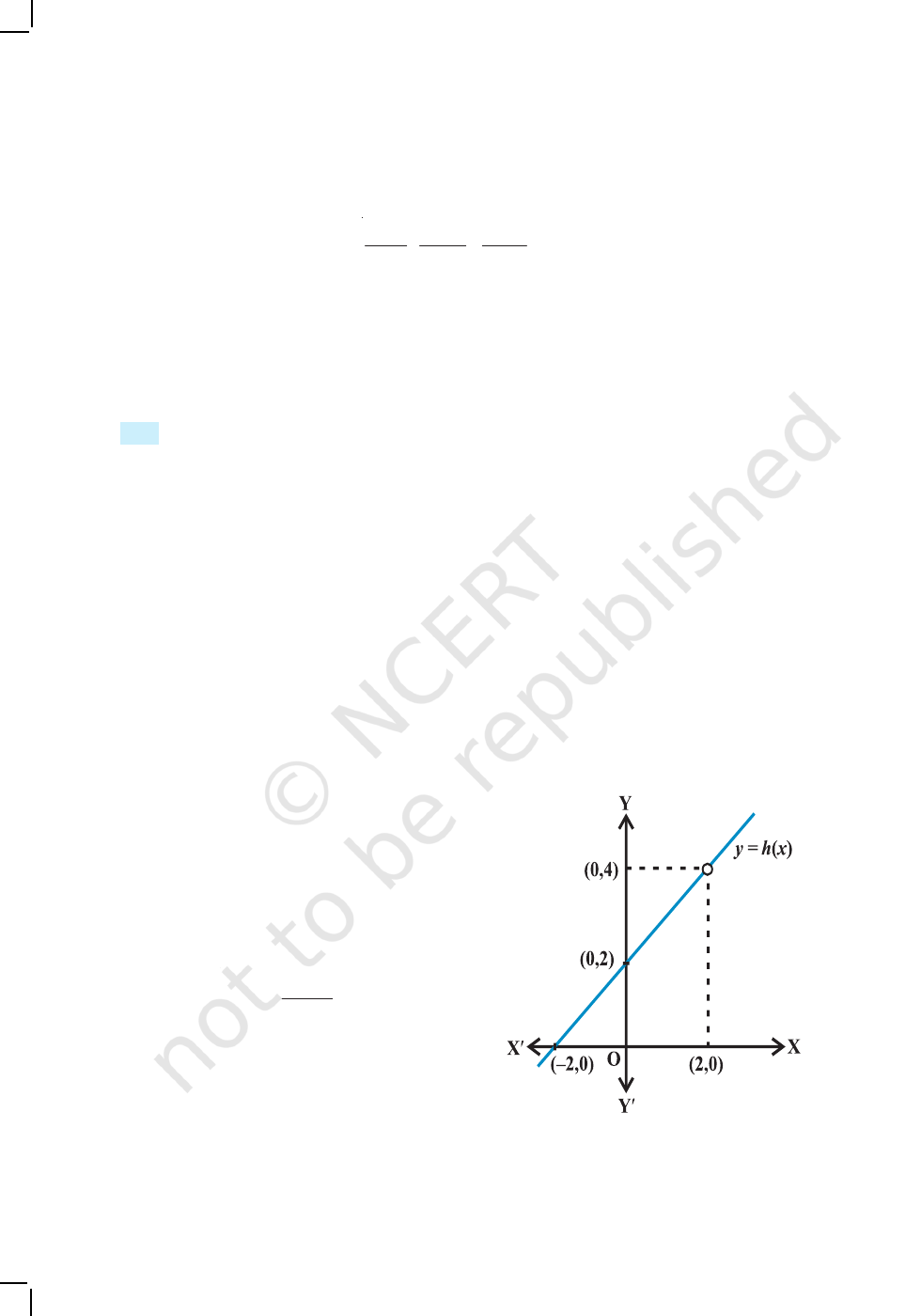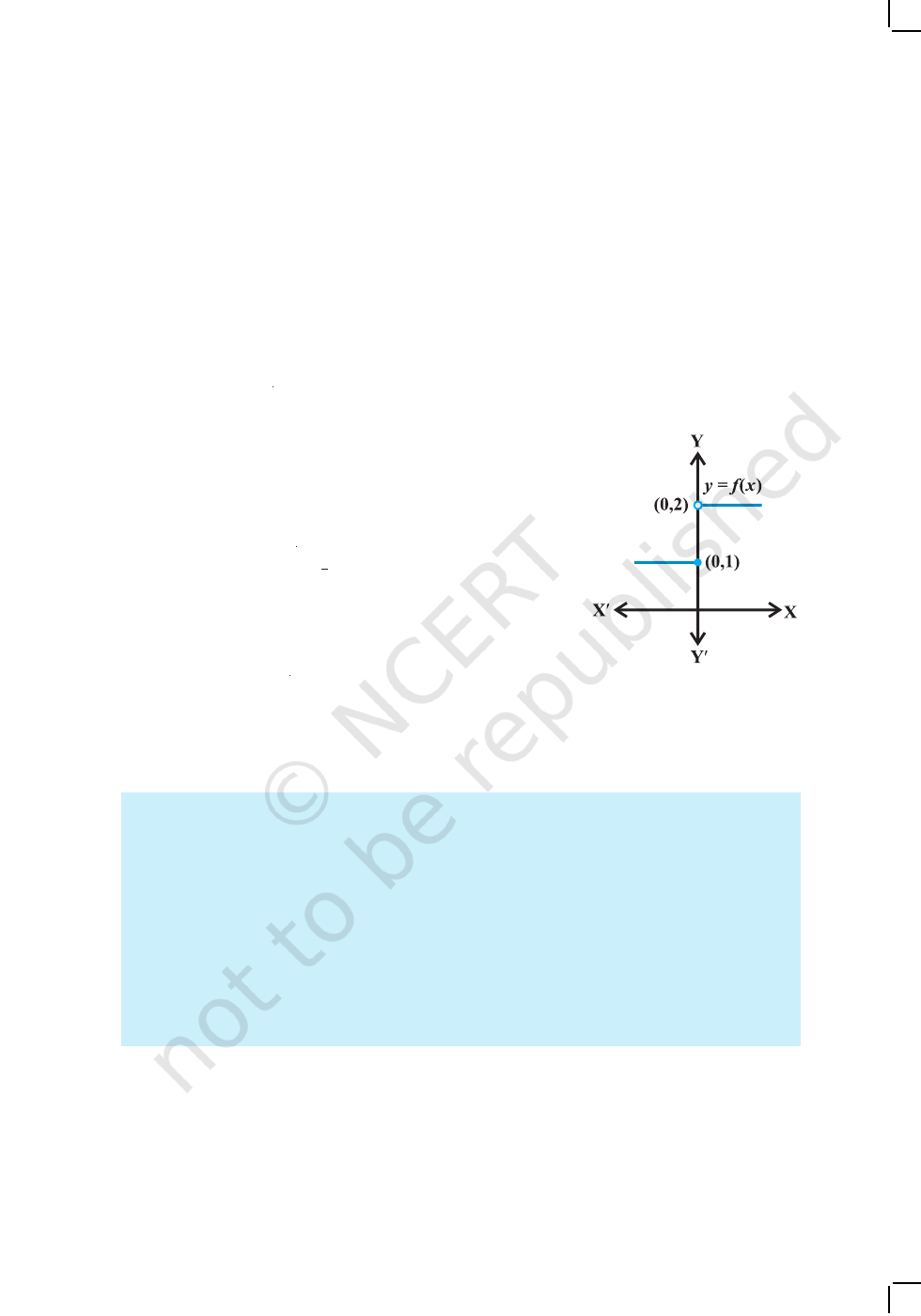vWith the Calculus as a key, Mathematics can be successfully applied to the
explanation of the course of Nature – WHITEHEAD v
13.1 Introduction
This chapter is an introduction to Calculus. Calculus is that
branch of mathematics which mainly deals with the study
of change in the value of a function as the points in the
domain change. First, we give an intuitive idea of derivative
(without actually defining it). Then we give a naive definition
of limit and study some algebra of limits. Then we come
back to a definition of derivative and study some algebra
of derivatives. We also obtain derivatives of certain
standard functions.
13.2 Intuitive Idea of Derivatives
Physical experiments have confirmed that the body dropped
from a tall cliff covers a distance of 4.9t
2
metres in t seconds,
i.e., distance s in metres covered by the body as a function of time t in seconds is given
by s = 4.9t
2
.
The adjoining Table 13.1 gives the distance travelled in metres at various intervals
of time in seconds of a body dropped from a tall cliff.
The objective is to find the veloctiy of the body at time t = 2 seconds from this
data. One way to approach this problem is to find the average velocity for various
intervals of time ending at t = 2 seconds and hope that these throw some light on the
velocity at t = 2 seconds.
Average velocity between t = t
1
and t = t
2
equals distance travelled between
t = t
1
and t = t
2
seconds divided by (t
2
t
1
). Hence the average velocity in the first
two seconds
13Chapter
LIMITS AND DERIVATIVES
Sir Issac Newton
(1642-1727)
2020-21282 MATHEMATICS
=
2 1
2 1
Distance travelled between 2 0
Time interval ( )
t and t
t t
= =
=
(
)
( )
19.6 0
9.8 /
2 0
m
m s
s
=
.
Similarly, the average velocity between t = 1
and t = 2 is
(
)
m
s
= 14.7 m/s
Likewise we compute the average velocitiy
between t = t
1
and t = 2 for various t
1
. The following
Table 13.2 gives the average velocity (v), t = t
1
seconds and t = 2 seconds.
Table 13.2
t
1
0 1 1.5 1.8 1.9 1.95 1.99
v 9.8 14.7 17.15 18.62 19.11 19.355 19.551
From Table 13.2, we observe that the average velocity is gradually increasing.
As we make the time intervals ending at t = 2 smaller, we see that we get a better idea
of the velocity at t = 2. Hoping that nothing really dramatic happens between 1.99
seconds and 2 seconds, we conclude that the average velocity at t = 2 seconds is just
above 19.551m/s.
This conclusion is somewhat strengthened by the following set of computation.
Compute the average velocities for various time intervals starting at t = 2 seconds. As
before the average velocity v between t = 2 seconds and t = t
2
seconds is
=
2
2
Distance travelled between 2 seconds and
seconds
2
t
t
=
2
2
Distance travelled in seconds Distance
travelled in 2 seconds
2
t
t
t s
0
0
1 4.9
1.5 11.025
1.8 15.876
1.9 17.689
1.95 18.63225
2 19.6
2.05 20.59225
2.1 21.609
2.2 23.716
2.5 30.625
3 44.1
4 78.4
Table 13.1
2020-21LIMITS AND DERIVATIVES 283
=
2
2
Distance travelled in seconds 19.6
2
t
t
The following Table 13.3 gives the average velocity v in metres per second
between t = 2 seconds and t
2
seconds.
Table 13.3
t
2
4 3 2.5 2.2 2.1 2.05
2.01
v 29.4 24.5 22.05 20.58 20.09 19.845 19.649
Here again we note that if we take smaller time intervals starting at t = 2, we get
better idea of the velocity at t = 2.
In the first set of computations, what we have done is to find average velocities
in increasing time intervals ending at t = 2 and then hope that nothing dramatic happens
just before t = 2. In the second set of computations, we have found the average velocities
decreasing in time intervals ending at t = 2 and then hope that nothing dramatic happens
just after t = 2. Purely on the physical grounds, both these sequences of average
velocities must approach a common limit. We can safely conclude that the velocity of
the body at t = 2 is between 19.551m/s and 19.649 m/s. Technically, we say that the
instantaneous velocity at t = 2 is between 19.551 m/s and 19.649 m/s. As is
well-known, velocity is the rate of change of displacement. Hence what we have
accomplished is the following. From the given data of distance covered at various time
instants we have estimated the rate of
change of the distance at a given instant
of time. We say that the derivative of
the distance function s = 4.9t
2
at t = 2
is between 19.551 and 19.649.
An alternate way of viewing this
limiting process is shown in Fig 13.1.
This is a plot of distance s of the body
from the top of the cliff versus the time
t elapsed. In the limit as the sequence
of time intervals h
1
, h
2
, ..., approaches
zero, the sequence of average velocities
approaches the same limit as does the
sequence of ratios
Fig 13.1
2020-21284 MATHEMATICS
3 3
1 1 2 2
1 2 3
C BC B C B
, ,
AC AC AC
, ...
where C
1
B
1
= s
1
s
0
is the distance travelled by the body in the time interval h
1
= AC
1
,
etc. From the Fig 13.1 it is safe to conclude that this latter sequence approaches the
slope of the tangent to the curve at point A. In other words, the instantaneous velocity
v(t) of a body at time t = 2 is equal to the slope of the tangent of the curve s = 4.9t
2
at
t = 2.
13.3 Limits
The above discussion clearly points towards the fact that we need to understand limiting
process in greater clarity. We study a few illustrative examples to gain some familiarity
with the concept of limits.
Consider the function f(x) = x
2
. Observe that as x takes values very close to 0,
the value of f(x) also moves towards 0 (See Fig 2.10 Chapter 2). We say
(
)
0
lim 0
x
f x
=
(to be read as limit of f (x) as x tends to zero equals zero). The limit of f (x) as x tends
to zero is to be thought of as the value f (x) should assume at x = 0.
In general as x a, f (x) l, then l is called limit of the function f (x) which is
symbolically written as
(
)
lim
x a
f x l
=
.
Consider the following function g(x) = |x|, x
0. Observe that g(0) is not defined.
Computing the value of g(x) for values of x very
near to 0, we see that the value of g(x) moves
towards 0. So,
0
lim
x
g(x) = 0. This is intuitively
clear from the graph of y = |x| for x
0.
(See Fig 2.13, Chapter 2).
Consider the following function.
( )
2
4
, 2
2
x
h x x
x
=
.
Compute the value of h(x) for values of
x very near to 2 (but not at 2). Convince yourself
that all these values are near to 4. This is
somewhat strengthened by considering the graph
of the function y = h(x) given here (Fig 13.2).
Fig 13.2
2020-21LIMITS AND DERIVATIVES 285
In all these illustrations the value which the function should assume at a given
point x = a did not really depend on how is x tending to a. Note that there are essentially
two ways x could approach a number a either from left or from right, i.e., all the
values of x near a could be less than a or could be greater than a. This naturally leads
to two limits – the right hand limit and the left hand limit. Right hand limit
of a
function f(x) is that value of f(x) which is dictated by the values of f(x) when x tends
to a from the right. Similarly, the left hand limit. To illustrate this, consider the function
( )
1, 0
2, 0
x
f x
x
=
>
Graph of this function is shown in the Fig 13.3. It is
clear that the value of f at 0 dictated by values of f(x) with
x 0 equals 1, i.e., the left hand limit of f (x) at 0 is
0
lim ( ) 1
x
f x
=
.
Similarly, the value of f at 0 dictated by values of
f (x) with x > 0 equals 2, i.e., the right hand limit of f (x)
at 0 is
0
lim ( ) 2
x
f x
+
=
.
In this case the right and left hand limits are different, and hence we say that the
limit of f (x) as x tends to zero does not exist (even though the function is defined at 0).
Summary
We say
lim
x a
f(x) is the expected value of f at x = a given the values of f near
x to the left of a. This value is called the left hand limit of f at a.
We say
lim ( )
x a
f x
+
is the expected value of f at x = a given the values of
f near x to the right of a. This value is called the right hand limit of f(x) at a.
If the right and left hand limits coincide, we call that common value as the limit
of f(x) at x = a and denote it by
lim
x a
f(x).
Illustration 1 Consider the function f(x) = x + 10. We want to find the limit of this
function at x = 5. Let us compute the value of the function f(x) for x very near to 5.
Some of the points near and to the left of 5 are 4.9, 4.95, 4.99, 4.995. . ., etc. Values
of the function at these points are tabulated below. Similarly, the real number 5.001,
Fig 13.3
2020-21286 MATHEMATICS
5.01, 5.1 are also points near and to the right of 5. Values of the function at these points
are also given in the Table 13.4.
Table 13.4
From the Table 13.4, we deduce that value of f(x)
at x = 5 should be greater than
14.995 and less than 15.001 assuming nothing dramatic happens between x = 4.995
and 5.001. It is reasonable to assume that the value of the f(x) at x = 5 as dictated by
the numbers to the left of 5 is 15, i.e.,
(
)
5
lim 15
x
f x
=
.
Similarly, when x approaches 5 from the right, f(x) should be taking value 15, i.e.,
(
)
5
lim 15
x
f x
+
=
.
Hence, it is likely that the left hand limit of f(x) and the right hand limit of f(x) are
both equal to 15. Thus,
(
)
(
)
(
)
5
5 5
lim lim lim 15
x
x x
f x f x f x
+
= = =
.
This conclusion about the limit being equal to 15 is somewhat strengthened by
seeing the graph of this function which is given in Fig 2.16, Chapter 2. In this figure, we
note that as x approaches 5 from either right or left, the graph of the function
f(x) = x +10 approaches the point (5, 15).
We observe that the value of the function at x = 5 also happens to be equal to 15.
Illustration 2 Consider the function f(x) = x
3
. Let us try to find the limit of this
function at x = 1. Proceeding as in the previous case, we tabulate the value of f(x) at
x near 1. This is given in the Table 13.5.
Table 13.5
From this table, we deduce that value of f(x) at x = 1 should be greater than
0.997002999 and less than 1.003003001 assuming nothing dramatic happens between
x 0.9 0.99 0.999 1.001 1.01 1.1
f(x) 0.729 0.970299 0.997002999 1.003003001 1.030301 1.331
x 4.9 4.95 4.99 4.995 5.001 5.01 5.1
f(x) 14.9 14.95 14.99 14.995 15.001 15.01 15.1
2020-21